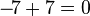# Opposite number facts for kids

Kids Encyclopedia Facts

In mathematics, opposite number or additive inverse of any number is a number n which, if added to n, results in 0 (the identity element of addition). The opposite number for n is written as −n. For example, −7 is the opposite number of 7, because$-\!7 + 7 = 0$.

For positive numbers, the opposite number is simply the negative version of the number. For negative numbers, the opposite number is the positive version of the number.

## Related pagesOpposite number Facts for Kids. Kiddle Encyclopedia.